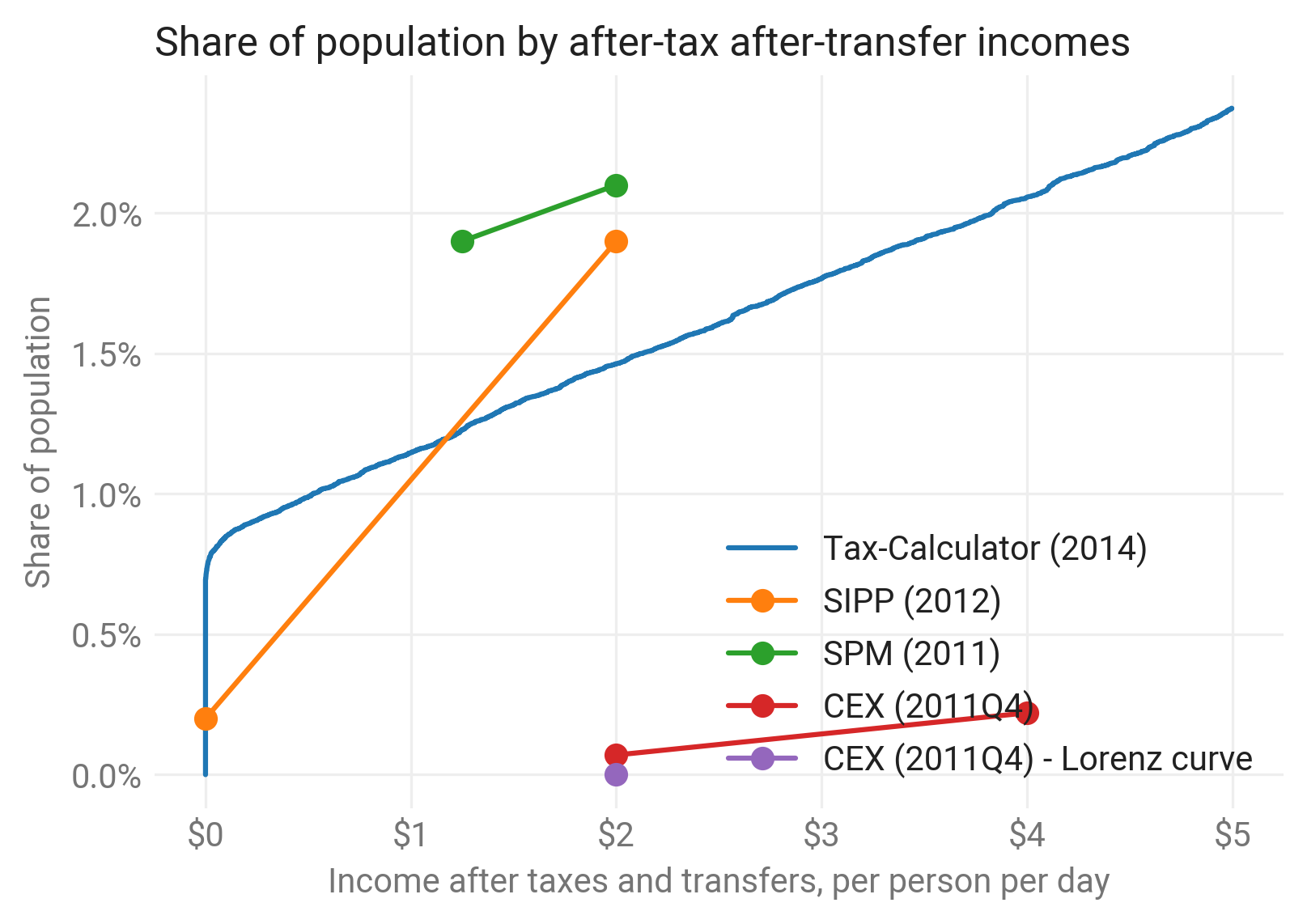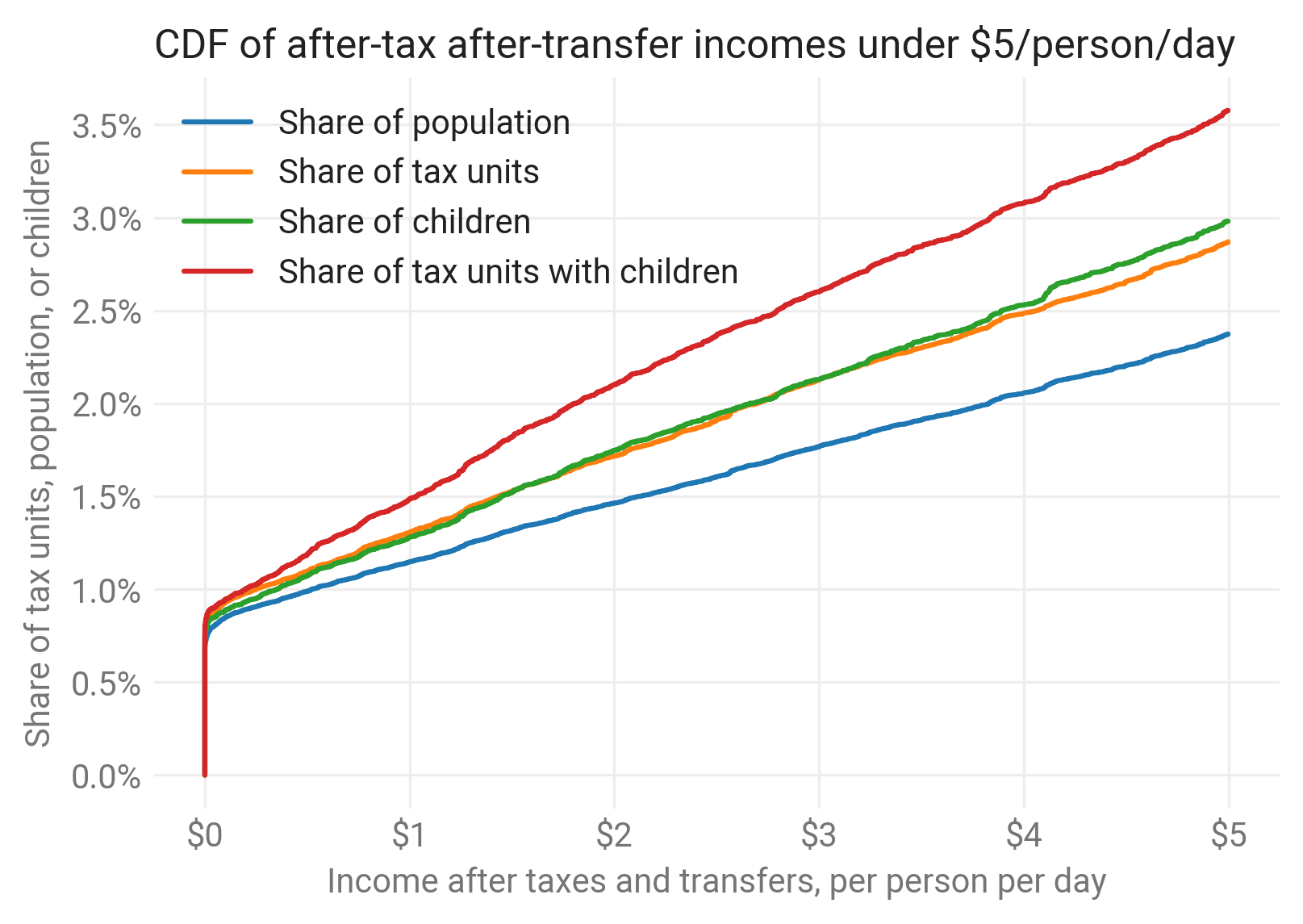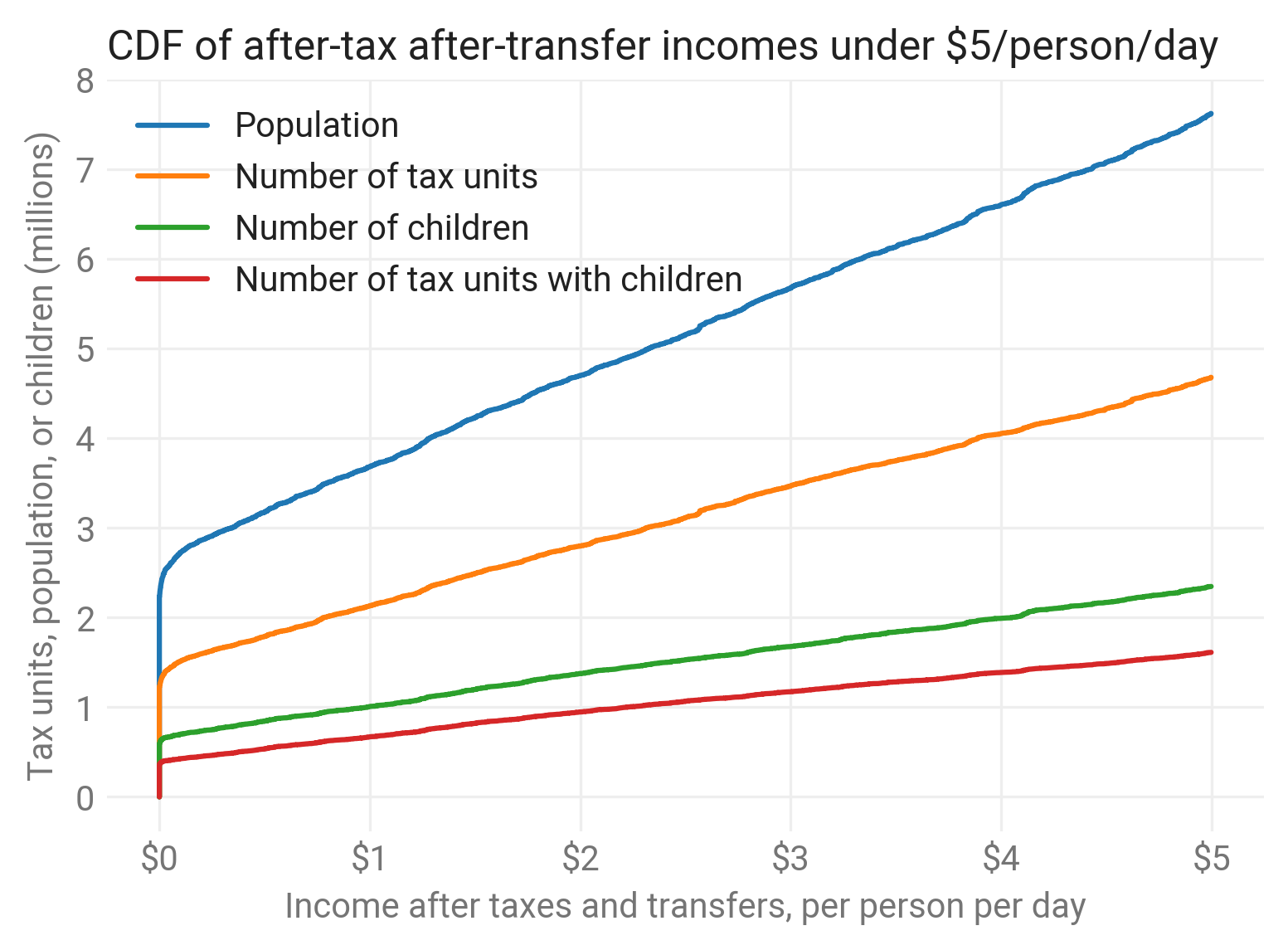Tax units with lowest after-tax after-transfer incomes¶

This notebook analyzes the distribution of tax units and individuals with the lowest after-tax after-transfer incomes. It compares Tax-Calculator's CPS + C-TAM data for the very bottom of the income scales, given the controversy around shares of Americans living in extreme poverty.

For example, Tax-Calculator estimates that 0.9M tax units with children have incomes below \$2 per person per day, and that these tax units include 1.4M children. http://www.twodollarsaday.com/ has said: the number of American families living on$2.00 per person, per day, has skyrocketed to one and a half million American households, including about three million children.

But this analysis has come under some criticism for not accounting for all transfer programs and time spent in poverty. Given Tax-Calculator results are annual, the primary comparison is against other annual surveys, as incomes can vary by month.

For example, Tax-Calculator says that 1.5% of the population is in tax units with total income under $2 per person per day. Depending on the source, consumption vs. income, and time period (not always known), other sources estimate this share between 0% and 2.1%. This notebook focuses on research from a Brookings Institution report examining these other sources. These other sources could be used to calibrate the C-TAM model. Data: CPS | Tax year: 2014 | Author: Max Ghenis | Date run: 2018-02-27 Setup¶ In : import taxcalc as tc import pandas as pd import numpy as np import matplotlib as mpl import matplotlib.pyplot as plt import seaborn as sns In : tc.__version__ Out: '0.16.1' In : sns.set_style('whitegrid') DPI = 300 mpl.rc('savefig', dpi=DPI) mpl.rcParams['figure.dpi']= DPI mpl.rcParams['font.sans-serif'] = 'Roboto' mpl.rcParams['font.family'] = 'sans-serif' # Set title text color to dark gray (https://material.io/color) not black. TITLE_COLOR = '#212121' mpl.rcParams['text.color'] = TITLE_COLOR # Axis titles and tick marks are medium gray. AXIS_COLOR = '#757575' mpl.rcParams['axes.labelcolor'] = AXIS_COLOR mpl.rcParams['xtick.color'] = AXIS_COLOR mpl.rcParams['ytick.color'] = AXIS_COLOR # Use Seaborn's default color palette. sns.set_palette(sns.color_palette()) Generate data¶ Tax-Calculator¶ Use 2014 data (i.e., don't advance_to_year) since comparison data points are in 2011-2012. In : recs = tc.Records.cps_constructor() calc = tc.Calculator(records=recs, policy=tc.Policy()) calc.calc_all() You loaded data for 2014. Tax-Calculator startup automatically extrapolated your data to 2014. Create DataFrame of the items desired. Which relevant columns are available? Totals, but really just care about != 0. In : df = calc.dataframe(['s006', 'aftertax_income', 'nu18', 'XTOT' ]) Other sources¶ Create DataFrames of other sources, in the order described in Smith and Chandy of Brookings. Survey of Income and Program Participation (SIPP): Initiated in 1976, the SIPP was specifically designed to overcome some of the known limitations of the Current Population Survey, the source of the official poverty rate, that disproportionately affect the estimation of incomes for poor households. These include the underreporting of participation in government welfare programs and unreliable estimates of irregular sources of income. Evidence confirms that the SIPP obtains the highest estimates of income among low-income households and the most accurate reporting of government program participation among comparable surveys. Smith and Chandy use 2012 SIPP data in their Brookings paper. Their first two charts show responses at a particular time, so are not comparable to annual Tax-Calculator data. This shows that data: In : # Estimated from Figure 1. sipp_snapshot = pd.DataFrame({'amount': [0, 1.25, 2], 'pop_share': [0.014, 0.026, 0.028]}) The 12-month estimates, which come from Figure 3 and this paragraph: Figure 3 illustrates this point. There is a dramatic decrease in those reporting no income and benefits when the reporting period is increased from one month to 12 months, as employed in the official U.S. poverty measure. This drives a reduction in our broad measure of$2 poverty: from 4 percent using survey responses over a single month, to 3 percent when responses are averaged over 4 months, to under 2 percent when responses are aggregated over a year.

I'll assume 1.9% where they say "under 2 percent."

In :
sipp = pd.DataFrame({'amount': [0, 2],
'pop_share': [0.002, 0.019]})

Supplemental Poverty Measure, described in Brookings (2011 data). It does not say what the period was, so this is unlikely to be a comparable 12-month estimate. I've asked on Chandy on Twitter here.

In :
spm = pd.DataFrame({'amount': [1.25, 2],
'pop_share': [0.019, 0.021]})

...which in contrast to the previous two surveys measures consumption as opposed to income, dates back to 1980 and is conducted principally for the purpose of computing weights of the Consumer Price Index.

Results are from this paragraph in Smith and Chandy:

Based on an assessment of consumption in the fourth quarter of 2011, we obtain a much lower $2 a day poverty rate of only 0.07 percent. and this CGD article: Thanks to rapid and generous sharing of data and code by Chandy and Smith, we’re able to use their analysis to see how many Americans are consumption poor at Deaton’s preferred$4 cutoff. The estimate is 0.22 percent compared to Deaton’s income-based estimate of 1.7 percent.

In :
ce = pd.DataFrame({'amount': [2, 4],
'pop_share': [0.0007, 0.0022]})

Chandy and Smith also use the World Bank approach of fitting a Lorenz curve to the distribution of responses to estimate poverty:

When we replicate this process using data from the U.S. CEX, our estimate of $2 a day poverty falls all the way to zero. In : ce_lorenz = pd.DataFrame({'amount': , 'pop_share': }) Preprocessing¶ Remove negatives. In : df = df[df['aftertax_income'] >= 0] Add after-tax income per person per day. In : df['aftertax_income_pppd'] = df['aftertax_income'] / (df['XTOT'] * 365) df['XTOT_s006'] = df['XTOT'] * df['s006'] df['nu18_s006'] = df['nu18'] * df['s006'] df['s006_with_children'] = np.where(df['nu18'] > 0, df['s006'], 0) Add % of total. In : df['XTOT_s006_pct'] = df['XTOT_s006'] / df['XTOT_s006'].sum() df['s006_pct'] = df['s006'] / df['s006'].sum() df['nu18_s006_pct'] = df['nu18_s006'] / df['nu18_s006'].sum() df['s006_with_children_pct'] = df['s006_with_children'] / df['s006_with_children'].sum() Add after-tax income percentile by sorting and summing cumsum of normalized weight. In : df.sort_values(by=['aftertax_income_pppd'], inplace=True) df['XTOT_s006_cumsum'] = df['XTOT_s006'].cumsum() df['s006_cumsum'] = df['s006'].cumsum() df['nu18_s006_cumsum'] = df['nu18_s006'].cumsum() df['s006_with_children_cumsum'] = df['s006_with_children'].cumsum() df['XTOT_s006_cumpct'] = df['XTOT_s006_pct'].cumsum() df['s006_cumpct'] = df['s006_pct'].cumsum() df['nu18_s006_cumpct'] = df['nu18_s006_pct'].cumsum() df['s006_with_children_cumpct'] = df['s006_with_children_pct'].cumsum() Analysis¶ Per person, compared to other sources¶ In : ax = df[df['aftertax_income_pppd'] <= 5].plot( x='aftertax_income_pppd', y='XTOT_s006_cumpct') sipp.plot(x='amount', y='pop_share', marker='o', ax=ax) spm.plot(x='amount', y='pop_share', marker='o', ax=ax) ce.plot(x='amount', y='pop_share', marker='o', ax=ax) ce_lorenz.plot(x='amount', y='pop_share', marker='o', ax=ax) ax.legend(['Tax-Calculator (2014)', 'SIPP (2012)', 'SPM (2011)', 'CEX (2011Q4)', 'CEX (2011Q4) - Lorenz curve']) ax.grid(color='#eeeeee') sns.despine(left=True, bottom=True) ax.set(xlabel='Income after taxes and transfers, per person per day', ylabel='Share of population') ax.xaxis.set_major_formatter(mpl.ticker.FuncFormatter(lambda x, _: '$' + format(int(x))))
ax.yaxis.set_major_formatter(mpl.ticker.FuncFormatter(lambda y, _: '{:.1%}'.format(y)))
plt.title('Share of population by after-tax after-transfer incomes', loc='left')
plt.show()Per tax unit, person, and child¶

Absolute counts and shares of total.

TODO: Avoid warning (https://github.com/pandas-dev/pandas/issues/18764).

In :
ax = df[df['aftertax_income_pppd'] <= 5].plot(
x='aftertax_income_pppd',
y=['XTOT_s006_cumpct', 's006_cumpct', 'nu18_s006_cumpct', 's006_with_children_cumpct'])
ax.legend(['Share of population', 'Share of tax units', 'Share of children',
'Share of tax units with children'])
ax.grid(color='#eeeeee')
sns.despine(left=True, bottom=True)
ax.set(xlabel='Income after taxes and transfers, per person per day',
ylabel='Share of tax units, population, or children')
ax.xaxis.set_major_formatter(mpl.ticker.FuncFormatter(lambda x, _: '$' + format(int(x)))) ax.yaxis.set_major_formatter(mpl.ticker.FuncFormatter(lambda y, _: '{:.1%}'.format(y))) plt.title('CDF of after-tax after-transfer incomes under$5/person/day', loc='left')
plt.show()
/usr/local/google/home/mghenis/miniconda2/lib/python2.7/site-packages/pandas/plotting/_core.py:1716: UserWarning: Pandas doesn't allow columns to be created via a new attribute name - see https://pandas.pydata.org/pandas-docs/stable/indexing.html#attribute-access
series.name = labelIn :
ax = df[df['aftertax_income_pppd'] <= 5].plot(
x='aftertax_income_pppd',
y=['XTOT_s006_cumsum', 's006_cumsum', 'nu18_s006_cumsum', 's006_with_children_cumsum'])
ax.legend(['Population', 'Number of tax units', 'Number of children',
'Number of tax units with children'])
ax.grid(color='#eeeeee')
sns.despine(left=True, bottom=True)
ax.set(xlabel='Income after taxes and transfers, per person per day',
ylabel='Tax units, population, or children (millions)')
ax.xaxis.set_major_formatter(mpl.ticker.FuncFormatter(lambda x, _: '$' + format(int(x)))) ax.yaxis.set_major_formatter(mpl.ticker.FuncFormatter(lambda y, _: (y / 1e6).astype(int))) plt.title('CDF of after-tax after-transfer incomes under$5/person/day', loc='left')
plt.show()Tables¶

Specific thresholds: \$2/day and \$4/day.

In :
(df.loc[df['aftertax_income_pppd'] <= 2,
['s006', 'XTOT_s006', 'nu18_s006', 's006_with_children']].sum() / 1e6).round(1)
Out:
s006                  2.8
XTOT_s006             4.7
nu18_s006             1.4
s006_with_children    0.9
dtype: float64
In :
(df.loc[df['aftertax_income_pppd'] <= 2,
['s006_pct', 'XTOT_s006_pct', 'nu18_s006_pct',
's006_with_children_pct']].sum() * 100).round(1)
Out:
s006_pct                  1.7
XTOT_s006_pct             1.5
nu18_s006_pct             1.7
s006_with_children_pct    2.1
dtype: float64
In :
(df.loc[df['aftertax_income_pppd'] <= 4,
['s006_pct', 'XTOT_s006_pct', 'nu18_s006_pct',
's006_with_children_pct']].sum() * 100).round(1)
Out:
s006_pct                  2.5
XTOT_s006_pct             2.1
nu18_s006_pct             2.5
s006_with_children_pct    3.1
dtype: float64